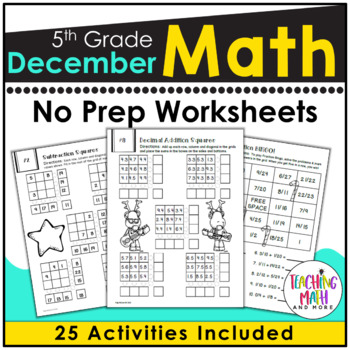# December Math Worksheets 5th Grade | Christmas Math Worksheets for 5th Grade5th
Standards
Resource Type
Formats Included
• PDF
Pages
56 pages

#### Also included in

1. This Math Worksheets Bundle is a COMPLETE 1-Year Bundle! The printables will keep your fifth graders having fun with math and be highly engaged! The worksheets have fun and challenging math problems. Not only are they PACKED with fifth-grade benchmark math problems, but they also give students fun c
\$60.00
\$93.50
Save \$33.50

### Description

Ready for 5th Grade Holiday Math? This December Math NO PREP packet that will keep your fifth graders engaged! This packet is just plain fun. Not only is it PACKED with fifth-grade math problems, it also gives students fun coloring, puzzles, and problem solving. Use this packet for bell work, classwork, extra credit, fast finishers, or homework! OR Use this packet for the long Winter Holiday break for students to stay engaged in Math!

FEATURES:

• 25 different math puzzle worksheets
• FUN activities and puzzles centered on reviewing math curriculum.
• Packed with 5th grade math problems for review and practice.
• A “tracking” page - as they complete days in the packet, students will color a part of a winter picture until they complete the packet.
• An ANSWER KEY for each worksheet.
• Lots of coloring fun. I’ve intentionally designed this packet with lots of pictures and borders to color. A MUST: Using a set of crayons or markers.

Topics Covered:

1. Use Four Operations to Solve
2. Factors & Multiples
4. Subtracting Decimals
5. Multiplying Decimals
6. Dividing Decimals
7. Comparing Fractions
8. Adding & Subtracting Fractions with unlike denominators
9. Multiplying Fractions

More December Math:

December NO PREP Math Packet - 3rd Grade

December NO PREP Math Packet - 4th Grade

Don't forget that leaving feedback earns you points toward FREE TPT purchases. I love that feedback!

Also, click HERE to follow me and be notified when new products are uploaded. New products are always on sale for the first 24 hours they are posted. It pays to follow me!

Thank you,

Kelly McCown

Total Pages
56 pages
Included
Teaching Duration
1 month
Report this Resource to TpT
Reported resources will be reviewed by our team. Report this resource to let us know if this resource violates TpT’s content guidelines.

### Standards

to see state-specific standards (only available in the US).
Apply and extend previous understandings of multiplication to multiply a fraction or whole number by a fraction.
Add and subtract fractions with unlike denominators (including mixed numbers) by replacing given fractions with equivalent fractions in such a way as to produce an equivalent sum or difference of fractions with like denominators. For example, 2/3 + 5/4 = 8/12 + 15/12 = 23/12. (In general, 𝘢/𝘣 + 𝘤/𝘥 = (𝘢𝘥 + 𝘣𝘤)/𝘣𝘥.)
Use parentheses, brackets, or braces in numerical expressions, and evaluate expressions with these symbols.
Add, subtract, multiply, and divide decimals to hundredths, using concrete models or drawings and strategies based on place value, properties of operations, and/or the relationship between addition and subtraction; relate the strategy to a written method and explain the reasoning used.
Explain patterns in the number of zeros of the product when multiplying a number by powers of 10, and explain patterns in the placement of the decimal point when a decimal is multiplied or divided by a power of 10. Use whole-number exponents to denote powers of 10.